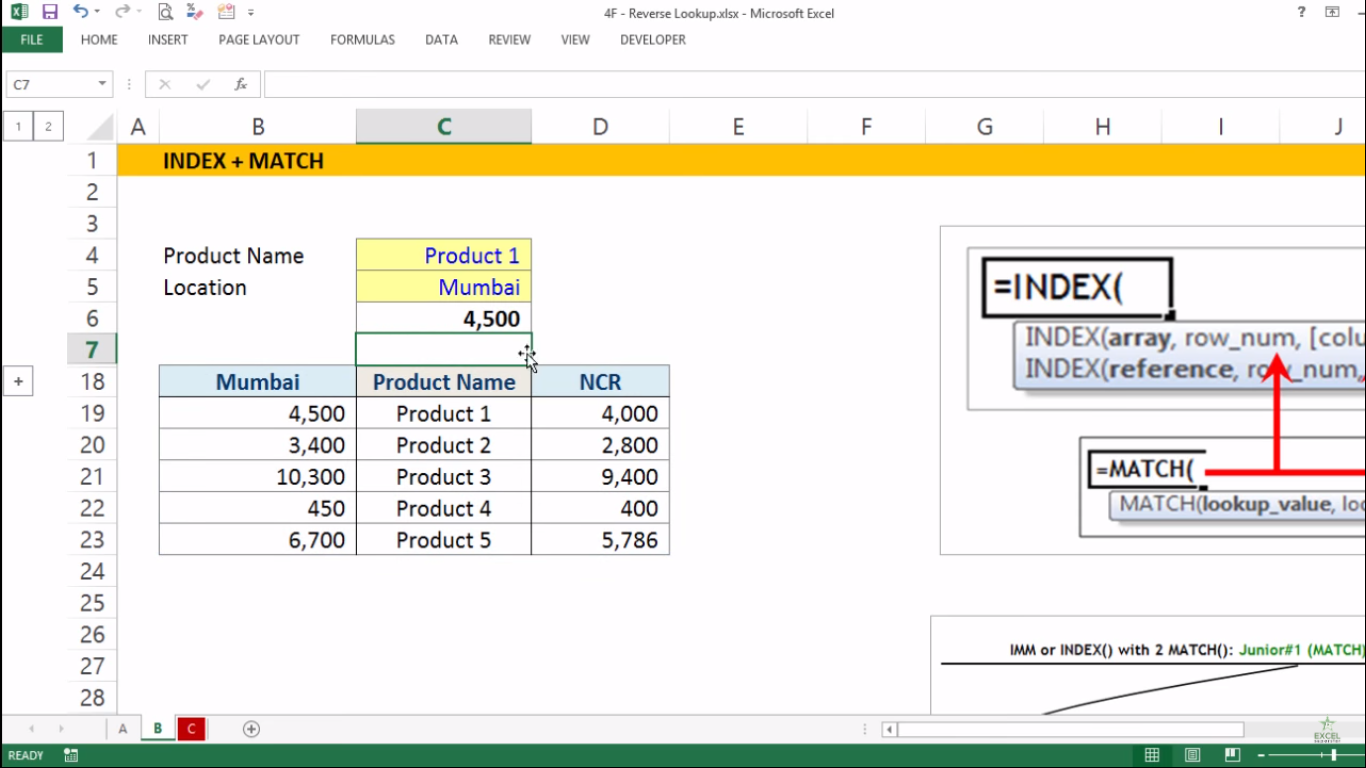# Excel Index + Match FormulaHello Everyone In this tutorial I will be teaching you the Basic of Index and Match Formula in Excel with the help of an example given below.

Example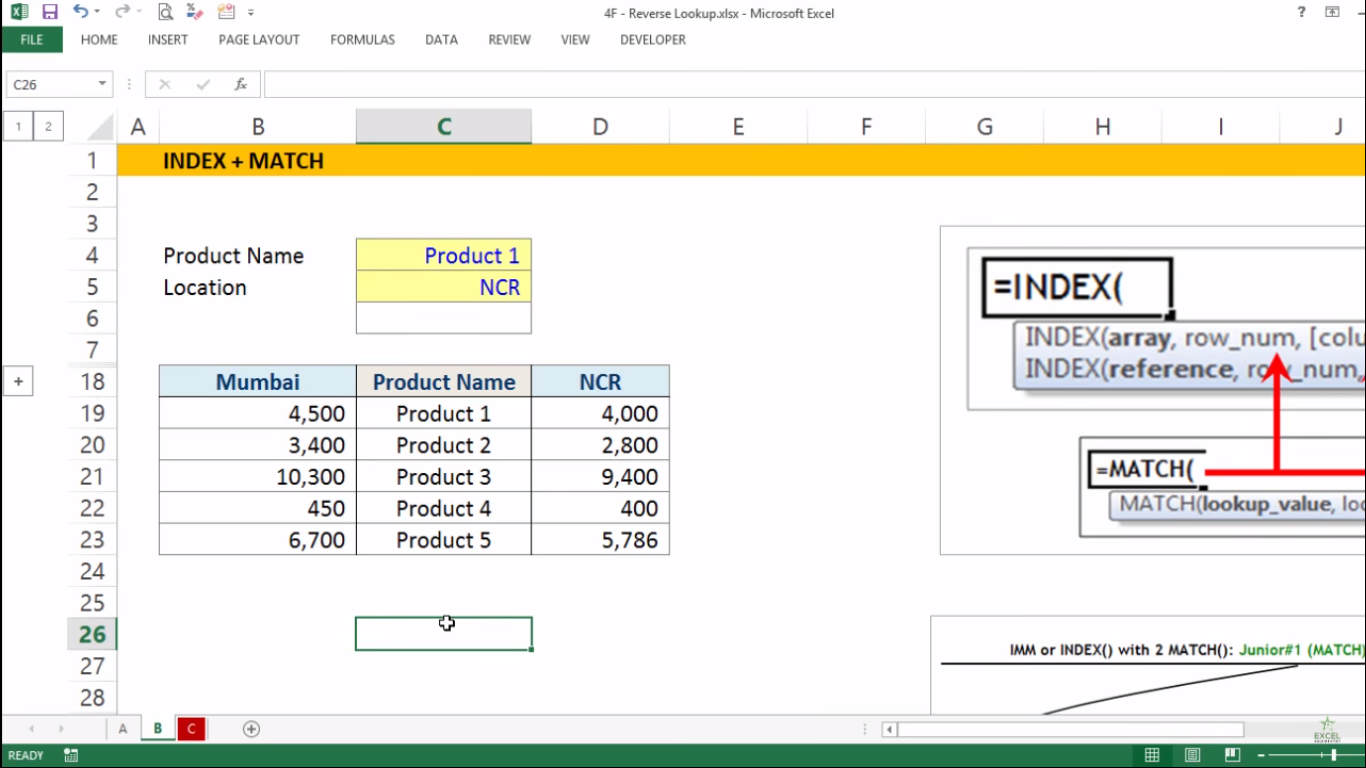Assume that you have a table that consists of Product Names along with the prices of Mumbai and NCR Region.

Now you have been asked that to choose the Product Name as Product 1 and Location as NCR in order to find out the price of the Product in the NCR Region.

So to get the answer here will be applying Index Formula by following the steps given below:

1. Write =INDEX and press the tab key =INDEX(Now there are 3 parameters (array, row_num, [column_num]).

The first parameter array means selecting the entire data inside the table

2. Choose the entire table and press F4 along with comma =INDEX(
\$B\$18: \$D\$23,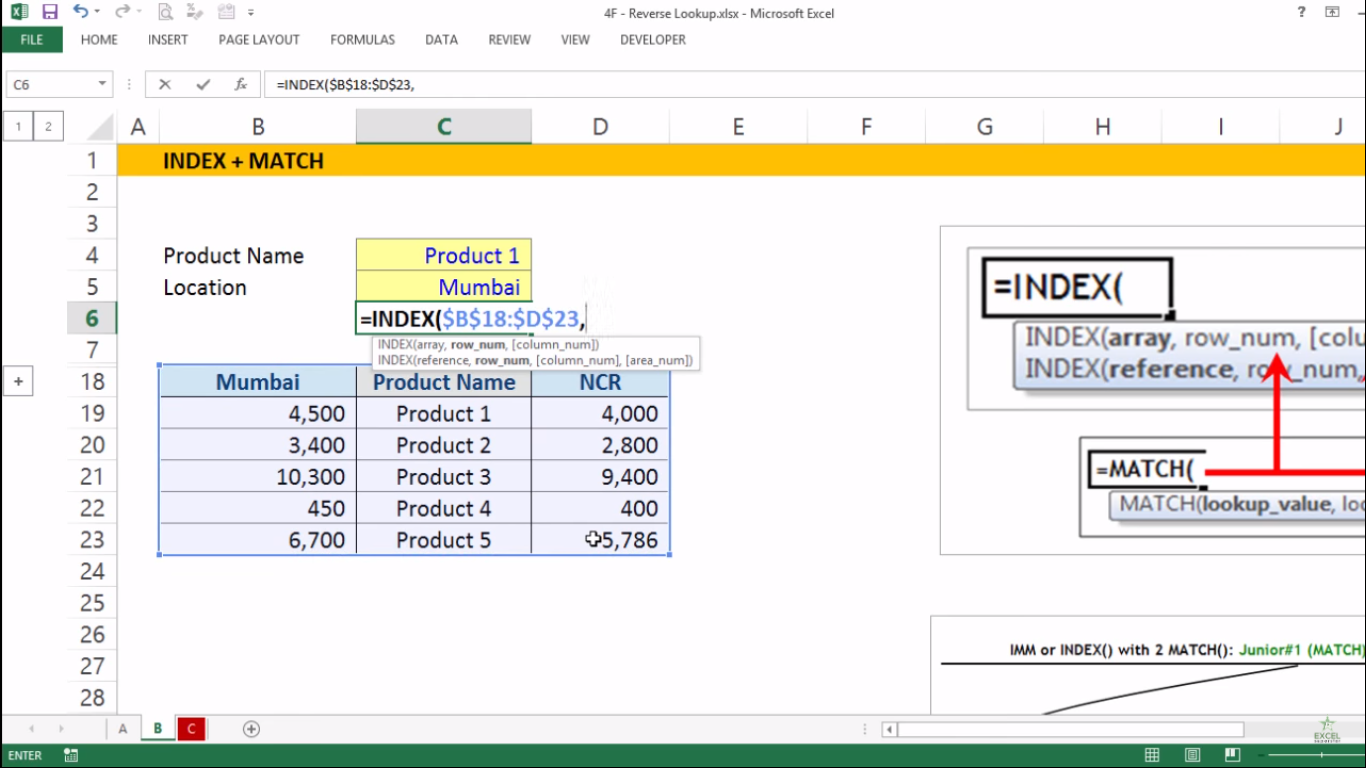The second parameter row_num means the row number in the array from which to return a value

3. So write 2 =INDEX( \$B\$18: \$D\$23,2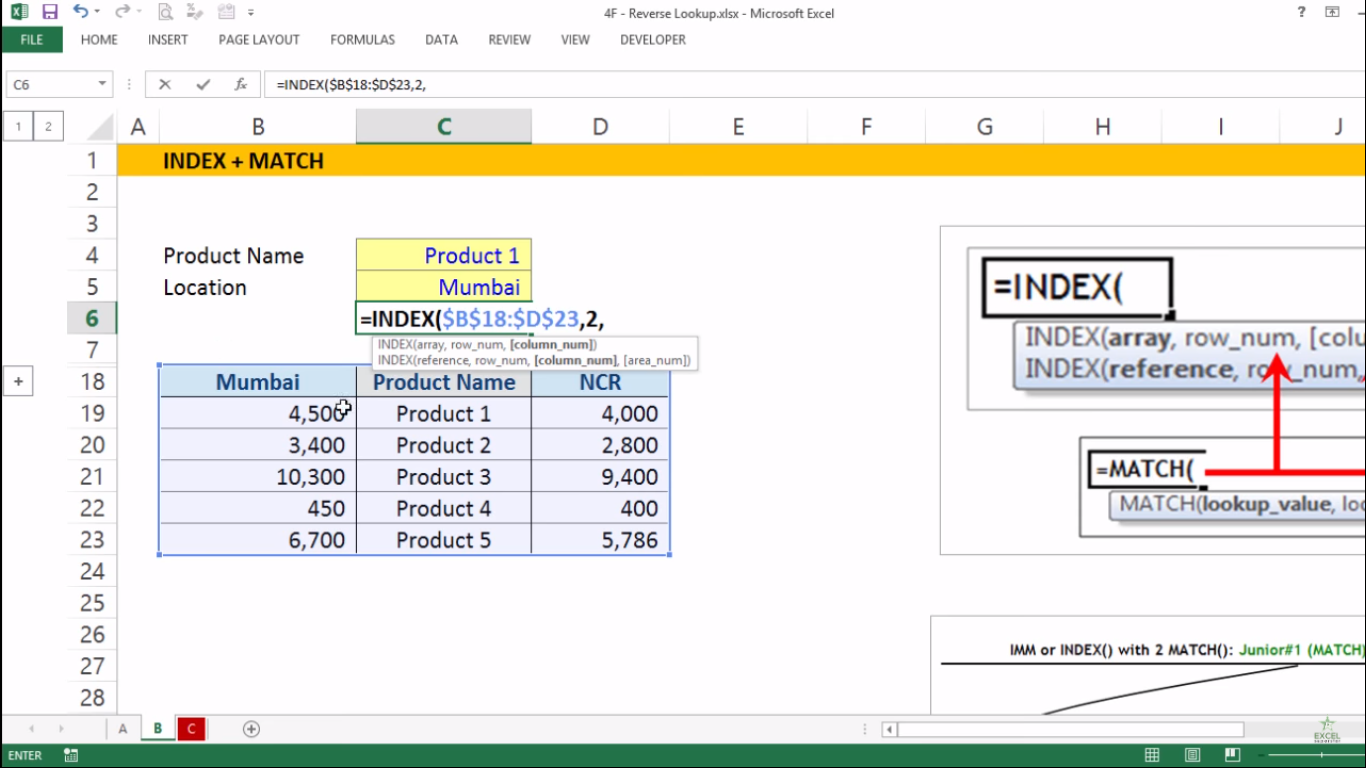Note – Here we are writing 2 because the table is talking about Product 1 which lies in the second Row

The second parameter [column_num] means the column number from which to return a value

4. So write 1 and close the bracket =INDEX( \$B\$18: \$D\$23,2,1)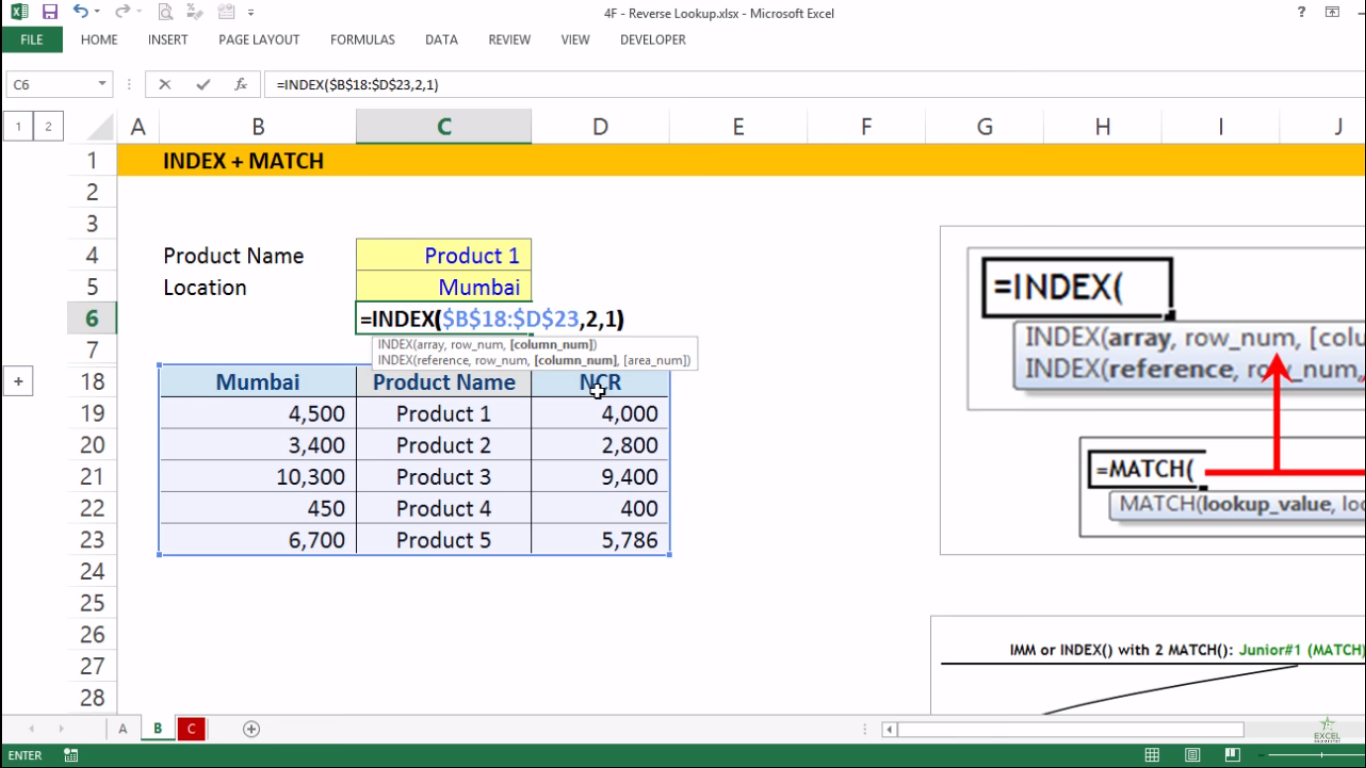Note – Here we are writing 1 because the prices of Mumbai is been asked so we will choose the first column from the table

5. Lastly, press Enter and you will get your answer as 4,500 which is the price of Product 1 for Mumbai Region